A colleague asked me an interesting question:

I have a journal article that includes sample quantiles for a variable. Given a new data value, I want to approximate its quantile. I also want to simulate data from the distribution of the published data. Is that possible?

This situation is common. You want to run an algorithm on published data, but you don't have the data. Even if you attempt to contact the original investigators, the data are not always available. The investigators might be dead or retired, or the data are confidential or proprietary. If you cannot obtain the original data, one alternative is to simulate data based on the published descriptive statistics.

Simulating data based on descriptive statistics often requires making some assumptions about the distribution of the published data. My colleague had access to quantile data, such as might be produced by the UNIVARIATE procedure in SAS. To focus the discussion, let's look at an example. The table to the left shows some sample quantiles of the total cholesterol in 35 million men aged 40-59 in the years 1999–2002. The data were published as part of the NHANES study.

With 35 million observations, the empirical cumulative distribution function (ECDF) is a good approximation to the distribution of cholesterol in the entire male middle-aged population in the US. The published quantiles only give the ECDF at 11 points, but if you graph these quantiles and connect them with straight lines, you obtain an approximation to the empirical cumulative distribution function, as shown below:My colleague's first question is answered by looking at the plot. If a man's total cholesterol is 200, you can approximate the quantile by using linear interpolation between the known values. For 200, the quantile is about 0.41. If you want a more exact computation, you can use a linear interpolation program.

The same graph also suggest a way to simulate data based on published quantiles of the data. The graph shows an approximate ECDF by using interpolation to "connect the dots" of the quantiles. You can use the inverse CDF method for simulation to simulate data from this approximate ECDF. Here are the main steps for the simulation:

• Use interpolation (linear, cubic, or spline) to model the ECDF. For a large sample of a continuous variable, the approximate ECDF will be a monotonic increasing function from the possible range of the variable X onto the interval [0,1].
• Generate N random uniform values in [0,1]. Call these values u1, u2, ..., uN.
• Because the approximate ECDF is monotonic, for each value ui in [0,1] there is a unique value of x (call it xi) that is mapped onto ui. For cubic interpolation or spline interpolation, you need to use a root-finding algorithm to obtain each xi. For linear interpolation, the inverse mapping is also piecewise linear, so you can use a linear interpolation program to solve directly for xi.
• The N values x1, x2, ..., xN are distributed according to the approximate ECDF.

The following graph shows the distribution of 10,000 simulated values from the piecewise linear approximation of the ECDF. The following table compares the quantiles of the simulated data (the SimEst column) to the quantiles of the NHANES data. The quantiles are similar except in the tails. This is typical behavior because the extreme quantiles are more variable than the inner quantiles. You can download the SAS/IML program that simulates the data and that creates all the images in this article.A few comments on this method:

• The more quantiles there are, the better the ECDF is approximated by the function that interpolate the quantiles.
• Extreme quantiles are important for capturing the tail behavior.
• The simulated data will never exceed the range of the original data. In particular, the minimum and maximum value of the sample determine the range of the simulated data.
• Sometimes the minimum and maximum values of the data are not published. For example, the table might end begin with the 0.05 quantile and end with the 0.95 quantile. In this case, you have three choices:
• Assign missing values to the 10% of the simulated values that fall outside the published range. They simulated data will be from a truncated distribution of the data. The simulated quantiles will be biased.
• Use interpolation to estimate the minimum and maximum values of the data. For example, you could use the 0.90 and 0.95 quantiles to extrapolate a value for the maximum data value.
• Use domain-specific knowledge to supply reasonable values for the minimum and maximum data values. For example, if the data are positive, you could use 0 as a lower bound for the minimum data value.If you’re an analyst, you know discovery in a complicated data set is one of the toughest problems to solve. But did you know the Business Knowledge Series course, Exploratory Analysis for Large and Complex Problems Using SAS Enterprise Miner, can help you solve those issues by tackling real-world problems? […]A colleague sent me an interesting question: What is the best way to abort a SAS/IML program? For example, you might want to abort a program if the data is singular or does not contain a sufficient number of observations or variables.

As a first attempt would be to try to use the ABORT statement, which is discussed in the article "how to stop processing your SAS/IML program if a certain condition is met". The following program contains a subtle error in logic:

```proc iml; ErrorFlag = 1; if ErrorFlag then ABORT; /* does not work as intended */ print "Execute statement 1"; print "Execute statement 2"; quit;```

This approach does not work because the ABORT statement terminate the IML procedure, but then SAS continues to process the next statement, which happens to be a PRINT statement. Since a PRINT statement is not a valid global SAS statement, an error occurs.

`ERROR 180-322: Statement is not valid or it is used out of proper order.`

### Use DO blocks or reverse the logic

The obvious solution is to use an ELSE statement to specify the conditional code to execute within PROC IML. At parse time, the PROC IML parser will process all DO blocks of an IF-THEN/ELSE statement. However, at run time only the DO block for the "true" condition will be executed. Consequently, the following statements parse and run without error:

```if ErrorFlag then ABORT; else do; print "Execute statement 1"; print "Execute statement 2"; end;```

An alternative solution is to forget about using the ABORT statement and reverse the conditional logic:

```ErrorFlag = 1; if ^ErrorFlag then do; print "Execute statement 1"; print "Execute statement 2"; end; quit;```

In this alternative solution, the PRINT statements are executed if the ErrorFlag variable is zero; otherwise they are not. In either case, the QUIT statement is the first executable statement after the IF-THEN/ELSE statement, so the procedure ends.

### Use modules

In a complex analysis, defining a module is a good way to encapsulate a task, be it validating data or computing with the validated data. For example, suppose your algorithm requires data that has at least three variables and no missing values. The following program divides the error checking task and the computational task between two modules:

```start CheckData(x); if ncol(x) < 3 then return(1); /* error */ if countmiss(x) > 0 then return(2); /* error */ return(0); /* no error */ finish;   start MyComputation(x); /* only called on validated data */ print "Execute statement 1"; print "Execute statement 2"; finish;   /* read x; validate data properties */ use MyData; read all var _num_ into x; close MyData;   ErrorFlag = CheckData(x); if ^ErrorFlag then run MyComputation(x); quit;```

With regards to defining modules, I'll also mention that you can use the RETURN statement anywhere in a module. This means that if you encounter a situation in the MyComputation module that requires you to abort the computation, you can use the RETURN statement to leave the module. Consequently, modules offer a robust mechanism for aborting a computation if an error condition occurs.

### Conclusions

There are other ways to organize your program so that statements can conditionally execute if there is an error. However, I will stop here. Do you have a favorite way to handle errors in PROC IML? Leave a comment.My last blog post described three ways to add a smoothing spline to a scatter plot in SAS. I ended the post with a cautionary note:

From a statistical point of view, the smoothing spline is less than ideal because the smoothing parameter must be chosen manually by the user.

This means that you can specify a smoothing spline parameter that appears to fit the data, but the selection is not based on an objective criterion. In contrast, SAS software supports several other methods for which the software can automatically choose a smoothing parameter that maximizes the goodness of fit while avoiding overfitting. This article describes penalized B-splines loess curves, and thin-plate splines.

### Penalized B-splines

You can fit penalized B-splines to data by using the TRANSREG procedure or by using the PBSPLINE statement in the SGPLOT procedure. The TRANSREG procedure enables you to specify many options that control the fit, including the criterion used to select the optimal smoothing parameter. The TRANSREG procedure supports the corrected Akaike criterion (AICC), the generalized cross-validation criterion (GCV), and Schwarz’s Bayesian criterion (SBC), among others. These criteria try to produce a curve that fits the data well, but does not interpolate or overfit the data. The default criterion is the AICC.

To illustrate fitting a smooth curve to a scatter plot, I'll use the SasHelp.enso data set, which contains data on the "southern oscillation," a cyclical phenomenon in atmospheric pressure that is linked to the El Niño and La Niña temperature oscillations in the Pacific Ocean. (ENSO is an acronym for El Niño-Southern Oscillation.) The ENSO data is analyzed in an example in the PROC TRANSREG documentation.

The following statements fit a penalized B-spline to the ENSO data:

```proc transreg data=sashelp.enso; model identity(pressure) = pbspline(year); /* AICC criterion */ run;```The data shows an oscillation of pressure in a yearly cycle. There are 14 peaks and valleys in this 14-year time series, which correspond to 14 winters and 14 summers.

### Loess curves

A loess curve is not a spline. A loess model at x uses a local neighborhood of x to compute a weighted least squares estimate. The smoothing parameter is the proportion of data in the local neighborhood: a value near 0 results in a curve that nearly interpolates the data whereas a value near 1 is nearly a straight line. You can fit loess curves to data by using the LOESS procedure or by using the LOESS statement in the SGPLOT procedure. The LOESS procedure enables you to specify many options that control the fit, including the criterion used to select the optimal smoothing parameter. The LOESS procedure supports the AICC and GCV criteria, among others. The default criterion is the AICC.

The default loess fit to the ENSO data is computed as follows:

```ods select FitSummary CriterionPlot FitPlot; proc loess data=sashelp.enso; model pressure = year; run;```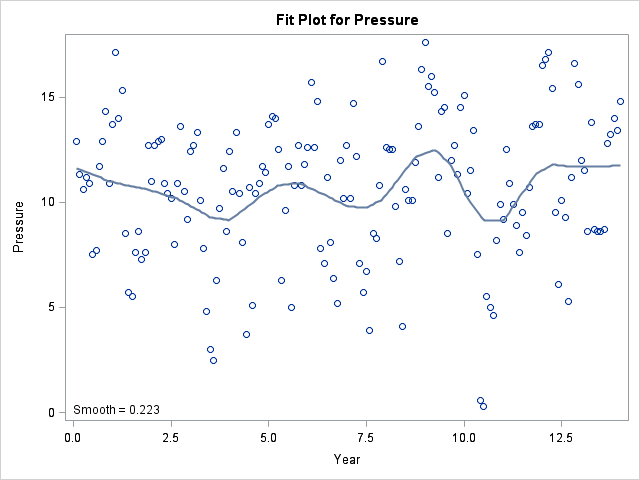The loess curve looks different than the penalized B-spline curve. The loess curve seems to contain irregularly spaced peaks that are 4–7 years apart. This is the El Niño oscillation cycle, which is an irregular cycle. The selected smoothing parameter is about 0.2, which means that about one-fifth of the observations are used for each local neighborhood during the estimation.

Earlier I said that the LOESS and TRANSREG procedures automatically choose parameter values that optimize some criterion. So why the difference in these plots? The answer is that the criterion curves sometimes have local minima, and for the ENSO data the LOESS procedure found a local minimum that corresponds to the El Niño cycle. The curve that shows the AICC criterion as a function of the smoothing parameter is shown below:The LOESS procedure supports several options that enable you to explore different ranges for the smoothing parameter or to search for a global minimum. I recommend using the PRESEARCH option in order to increase the likelihood of finding a global minimum. For example, for the ENSO data, the following call to PROC LOESS finds the global minimum at 0.057 and produces a scatter plot smoother that looks very similar to the penalized B-spline smoother.

```proc loess data=sashelp.enso; model pressure = year / select=AICC(presearch); run;```

### Smoothers with the SGPLOT procedure

Because the loess and penalized B-spline smoothers work well for most data, the SGPLOT procedure supports the PBSPLINE and LOESS statements for adding smoothers to a scatter plot. By default the statements also add the scatter plot markers, so use the NOMARKERS options if you are overlaying a smoother on an existing scatter plot:

```proc sgplot data=sashelp.enso; pbspline x=year y=pressure; /* also draws scatter plot */ loess x=year y=pressure / nomarkers; /* uses PRESEARCH option */ run;```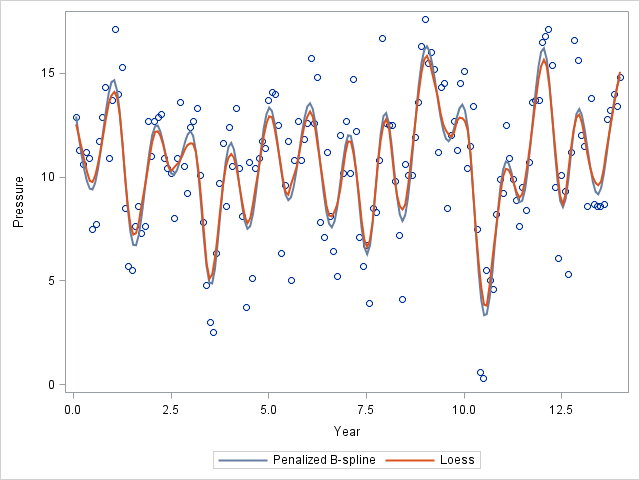As you can see, the two smoothers produce very similar results on the ENSO data. That is often the case, but not always.

### Thin-plate splines

I'm not going to shows examples of every nonparametric regression procedure in SAS, but I do want to mention one more. A thin-plate spline yet another spline that can be used to smooth data. The TPSPLINE procedure supports the GCV criterion for the automatic selection of the smoothing parameter. For the ENSO data, the TPSPLINE procedure produces a smoother the reveals the annual pressure cycle and is very similar to the previous curves:

```ods select FitStatistics CriterionPlot FitPlot; proc tpspline data=sashelp.enso; model pressure = (year); run;```

In conclusion, SAS software provides several ways to fit a smoother to data. The procedures mentioned in this article can automatically select a smoothing parameter that satisfies some objective criterion which balances goodness of fit with model parsimony. Do you have a favorite smoother? Let me know in the comments.In 2013 I published 110 blog posts. Some of these articles were more popular than others, often because they were linked to from a SAS newsletter such as the SAS Statistics and Operations Research News. In no particular order, here are some of my most popular posts from 2013, organized into categories. I've included two posts from December of 2012 because December articles rarely get a chance to appear on these "Best of" lists.

### Fun articles

Although I generally write about statistical topics and programming, sometimes I just like to have a little fun:

• Like fractals? Like Christmas? Then you will enjoy seeing the fractal Christmas tree that I created for Christmas 2012.
• Do you know how old your version of SAS is? Read the main article to discover the dates of SAS releases going back to SAS version 8.0, and read the comments to go back in time to 1972!

### The International Year of Statistics

To celebrate 2013 as the International Year of Statistics, I wrote several historical articles:

### Simulation

As the preceding titles indicate, often "popular" is correlated with "less technical." But not always. In 2013 I published Simulating Data with SAS, and several of my more popular articles dealt with how to efficiently carry out simulation with SAS software.

### Statistical Graphics and Data Analysis

Statistical data analysis and graphics are the "bread and butter" of my blog. Here are a few articles that attracted more readers than usual.

Start your new year by (re-)reading some of these popular posts from 2013. Next week I'll resume posting new articles on topics in statistics, programming, graphics, and simulation. Happy New Year to all my readers!The mosaic plot is a graphical visualization of a frequency table. In a previous post, I showed how to use the FREQ procedure to create a mosaic plot. This article shows how to create a mosaic plot by using the MOSAICPARM statement in the graph template language (GTL). (The MOSAICPARM statement was added in SAS 9.3m2.) The GTL gives you control over the characteristics of the plot, including how to color each tile.

### A basic template for a mosaic plot

The MOSAICPARM statement produces a mosaic plot from pre-summarized categorical data. Therefore, the first step is to specify a set of categories, and the frequencies for each category. I'll use the same Sashelp.Heart data set that I used in my previous post. You can download the program that specifies the order of levels for certain categorical variables. The following statements use PROC FREQ to summarize the table. Several additional statistics are also computed for each cell, such as the expected value (under the hypothesis of no association between blood pressure and weight) and the standardized residual (under the same model). The summary is written to the FreqOut data set, which is used to create the mosaic plot.

```/* summarize the data */ proc freq data=heart; tables BP_Cat*Weight_Cat / out=FreqOut(where=(Percent^=.)); run;   /* create basic mosaic plot with no tile colors */ proc template; define statgraph BasicMosaicPlot; begingraph; layout region; MosaicPlotParm category=(Weight_Cat BP_Cat) count=Count; endlayout; endgraph; end; run;   proc sgrender data=FreqOut template=BasicMosaicPlot; run;```The mosaic plot is the same was produced by PROC FREQ in my previous post, except that no colors are assigned to the cells. Also, PROC FREQ reverses the Y axis so that the mosaic plot is in the same order as the frequency table. See my previous post for how to interpret a mosaic plot.

### A template for a mosaic plot with custom cell colors

As I said, the GTL enables you to specify colors for the cells. All you need to do is to include a variable in the summary data set that species the color. You can specify a discrete palette of colors by using the COLORGROUP= option in the MOSAICPLOTPARM statement. Alternatively, you can specify a continuous spectrum of colors by using the COLORRESPONSE= option in the MOSAICPLOTPARM statement.

A clever use of colors is to color each cell in the mosaic plot by the residual (observed count minus expected count) of a hypothesized model (Friendly, 1999, JGCS). The simplest model is the "independence model," in which the expected count for each cell is simply the product of the marginal counts for each variable. (This is the null hypothesis for the chi-square test for independence.) In order to make the residuals comparable across cells, I will generate standardized residuals. The following PROC FREQ call adds standardized residuals and other statistics to the summary of the data. The summary is written to the FreqList data set, which is used to create the mosaic plot.

```proc freq data=heart; tables BP_Cat*Weight_Cat / norow cellchi2 expected stdres crosslist; ods output CrossList=FreqList(where=(Expected>0)); run;   /* color by response (notice that PROC FREQ reverses Y axis) */ proc template; define statgraph mosaicPlotParm; begingraph; layout region; MosaicPlotParm category=(Weight_Cat BP_Cat) count=Frequency / colorresponse=StdResidual name="mosaic"; continuouslegend "mosaic" / title="StdRes"; endlayout; endgraph; end; run;   proc sgrender data=FreqList template=mosaicPlotParm; run;```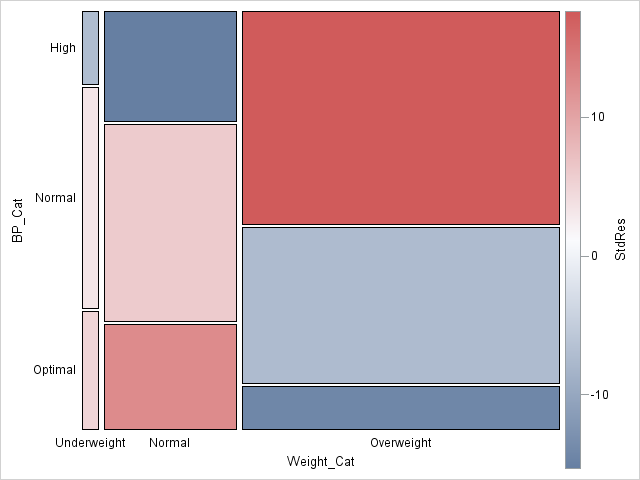Notice that I used the COLORRESPONSE= option on the MOSAICPLOTPARM statement to specify that each tile be colored according to the range of standardized residuals. The CONTINUOUSLEGEND statement adds a three-color ramp to the plot and automatically shows the association between colors and the standardized residuals.

From the mosaic plot, you can visually see why the null hypothesis (no association) is rejected for these data. Red is used for cells with large positive deviations from the no-association model, which means a higher-than-expected observed count. Blue is used for large negative residuals. Among the overweight patients, more have high blood pressure than would be expected by the no-association model.

### A generalized template for a mosaic plot

The previous template can be generalized in two ways:

• The template can use dynamic variables instead of hard-coding the variables for this particular medical study.
• The three-color ramp can be improved by making sure that the range is symmetric and that zero is exactly at the center of the color ramp.

The following improved and generalized template supports these two features. The resulting graph is not shown, since it is similar to the previous graph. However, this template is general enough to be used for a variety of data sets. The template is suitable for handling summaries that are produced by using the CROSSLIST option in the TABLES statement in PROC FREQ.

```/* adjust range of values for color ramp; add dynamic variables */ proc template; define statgraph mosaicPlotGen; dynamic _X _Y _Frequency _Response _Title _LegendTitle; /* dynamic vars */ begingraph; entrytitle _Title; /* make sure color ramp is symmetric */ rangeattrmap name="responserange" ; range negmaxabs - maxabs / rangecolormodel=ThreeColorRamp; endrangeattrmap ;   rangeattrvar attrvar=rangevar var=_Response attrmap="responserange"; layout region; MosaicPlotParm category=(_X _Y) count=_Frequency / name="mosaic" colorresponse=rangevar; continuouslegend "mosaic" / title=_LegendTitle; endlayout; endgraph; end; run;   proc sgrender data=FreqList template=mosaicPlotGen; dynamic _X="Weight_Cat" _Y="BP_Cat" _Frequency="Frequency" _Response="StdResidual" _Title="Blood Pressure versus Weight" _LegendTitle="StdResid"; run;```Mosaic plots (Hartigan and Kleiner, 1981; Friendly, 1994, JASA) are used for exploratory data analysis of categorical data. Mosaic plots have been available for decades in SAS products such as JMP, SAS/INSIGHT, and SAS/IML Studio. However, not all SAS customers have access to these specialized products, so I am pleased that mosaic plots have recently been added to two Base SAS procedures:

Both of these features were added in SAS 9.3m2, which is the 12.1 release of the analytics products. This article describes how to create a mosaic plot by using PROC FREQ. My next blog post will describe how to create mosaic plots by using the GTL.

### Use mosaic plots to visualize frequency tables

You can use mosaic plots to visualize the cells of a frequency table, also called a contingency table or a crosstabulation table. A mosaic plot consists of regions (called "tiles") whose areas are proportional to the frequencies of the table cells. The widths of the tiles are proportional to the frequencies of the column variable levels. The heights of tiles are proportional to the frequencies of the row levels within the column levels.

The FREQ procedure supports two-variable mosaic plots, which are the most important case. The GTL statement supports mosaic plots with up to three categorical variables. JMP and SAS/IML Studio enable you to create mosaic plots with even more variables.

As I showed in a previous blog post, you can use user-defined formats to specify the order of levels of a categorical variable. The Sashelp.Heart data set contains data for 5,209 patients in a medical study of heart disease. You can download the program that that specifies the order of levels for certain categorical variables. The following statements use the ordered categories to create a mosaic plot. The plot shows the relationship between categories of blood pressure and body weight for the patients:

```ods graphics on; proc freq data=heart; tables BP_Cat*Weight_Cat / norow chisq plots=MOSAIC; /* alias for MOSAICPLOT */ run;```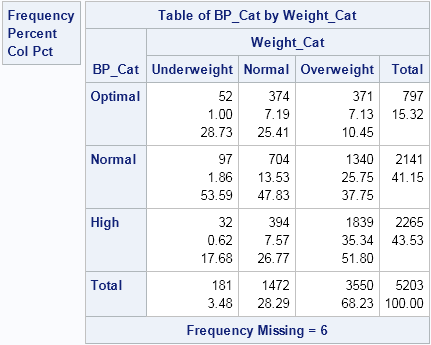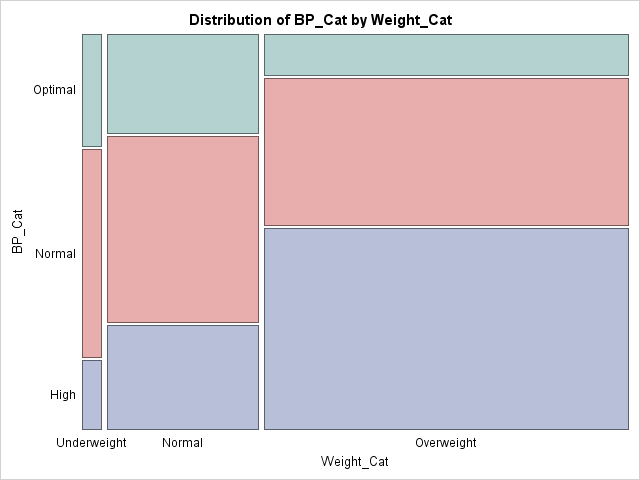The mosaic plot is a graphical depiction of the frequency table. The mosaic plot shows the distribution of the weight categories by dividing the X axis into three intervals. The length of each interval is proportional to the percentage of patients who are underweight (3.5%), normal weight (28%), and overweight (68%), respectively. Within each weight category, the patients are further subdivided. The first column of tiles shows the proportion of patients who have optimal (29%), normal (54%), or high (18%) blood pressure, given that they are underweight. The middle column shows similar information for the patients of normal weight. The last column shows the conditional distribution of blood pressure categories, given that the patients are overweight.

The chi-square test (not shown) tests the hypothesis that there is no association between the weight of patients and their blood pressure. The chi-square test rejects that hypothesis, and the mosaic plot shows why. If there were no association between the variables, the green, red, and blue tiles would be essentially the same height regardless of the weight category of the patients. They are not. Rather, the height of the blue tiles increases from left to right. This shows that high blood pressure is more prevalent in overweight patients. Similarly, the height of the green tiles decreases from left to right. This shows that optimal blood pressure occurs more often in normal and underweight patents than in overweight patients.

The colors in this mosaic plot indicate the levels of the second variable. This enables you to quickly assess how categories of that variable depend on categories of the first variable. There are other ways to color the mosaic plot tiles, and you can use the GTL to specify an alternate set of colors. I describe that approach in my next blog post.Mosaic plots (Hartigan and Kleiner, 1981; Friendly, 1994, JASA) are used for exploratory data analysis of categorical data. Mosaic plots have been available for decades in SAS products such as JMP, SAS/INSIGHT, and SAS/IML Studio. However, not all SAS customers have access to these specialized products, so I am pleased that mosaic plots have recently been added to two Base SAS procedures:

Both of these features were added in SAS 9.3m2, which is the 12.1 release of the analytics products. This article describes how to create a mosaic plot by using PROC FREQ. My next blog post will describe how to create mosaic plots by using the GTL.

### Use mosaic plots to visualize frequency tables

You can use mosaic plots to visualize the cells of a frequency table, also called a contingency table or a crosstabulation table. A mosaic plot consists of regions (called "tiles") whose areas are proportional to the frequencies of the table cells. The widths of the tiles are proportional to the frequencies of the column variable levels. The heights of tiles are proportional to the frequencies of the row levels within the column levels.

The FREQ procedure supports two-variable mosaic plots, which are the most important case. The GTL statement supports mosaic plots with up to three categorical variables. JMP and SAS/IML Studio enable you to create mosaic plots with even more variables.

As I showed in a previous blog post, you can use user-defined formats to specify the order of levels of a categorical variable. The Sashelp.Heart data set contains data for 5,209 patients in a medical study of heart disease. You can download the program that that specifies the order of levels for certain categorical variables. The following statements use the ordered categories to create a mosaic plot. The plot shows the relationship between categories of blood pressure and body weight for the patients:

```ods graphics on; proc freq data=heart; tables BP_Cat*Weight_Cat / norow chisq plots=MOSAIC; /* alias for MOSAICPLOT */ run;```The mosaic plot is a graphical depiction of the frequency table. The mosaic plot shows the distribution of the weight categories by dividing the X axis into three intervals. The length of each interval is proportional to the percentage of patients who are underweight (3.5%), normal weight (28%), and overweight (68%), respectively. Within each weight category, the patients are further subdivided. The first column of tiles shows the proportion of patients who have optimal (29%), normal (54%), or high (18%) blood pressure, given that they are underweight. The middle column shows similar information for the patients of normal weight. The last column shows the conditional distribution of blood pressure categories, given that the patients are overweight.

The chi-square test (not shown) tests the hypothesis that there is no association between the weight of patients and their blood pressure. The chi-square test rejects that hypothesis, and the mosaic plot shows why. If there were no association between the variables, the green, red, and blue tiles would be essentially the same height regardless of the weight category of the patients. They are not. Rather, the height of the blue tiles increases from left to right. This shows that high blood pressure is more prevalent in overweight patients. Similarly, the height of the green tiles decreases from left to right. This shows that optimal blood pressure occurs more often in normal and underweight patents than in overweight patients.

The colors in this mosaic plot indicate the levels of the second variable. This enables you to quickly assess how categories of that variable depend on categories of the first variable. There are other ways to color the mosaic plot tiles, and you can use the GTL to specify an alternate set of colors. I describe that approach in my next blog post.If you've ever tried to use PROC FREQ to create a frequency table of two character variables, you know that by default the categories for each variable are displayed in alphabetical order. A different order is sometimes more useful. For example, consider the following two-way table for the smoking status and weight status of 5,167 patients in a medical study, as recorded in the Sashelp.Heart data set, which is distributed with SAS software:

```proc freq data=sashelp.heart; tables Smoking_Status*Weight_Status / norow nocol nopct; run;```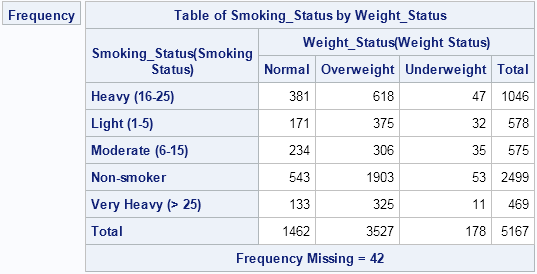The alphabetical order of the categories is not the best choice for these data. A better table would order the weight categories as "Underweight," "Normal," and "Overweight." Similarly, the smoking categories should be ordered from 'Non-smoker' to "Very Heavy (> 25)."

In most introductory SAS courses—and at almost every SAS conference—you will hear recommendations about how to encode ordinal variables when you create a SAS data set. Two common ways to make specify the order of categories are:

• Create (or sort) the data in the order that you want the frequency table to appear. Use the ORDER=DATA option on the PROC FREQ statement to instruct the procedure that it should order categories as they appear in the data set.
• Encode the data as a numerical variable with values 1, 2, 3, ..., and use a SAS format to provide the text that will be displayed for each category. The FREQ procedure will order the (numerical) variables by 1, 2, 3, ..., but will display the formatted values as the headers for the frequency tables.

The first approach is simple, but it has limitations. Often the data set is handed to you; you don't get to create it. You can use PROC SORT to create a sorted copy of the data, but you still need to provide variables with values such as 1, 2, 3, ..., in order to sort the data. Furthermore, if some joint categories are empty (for example, there are no underweight non-smokers in the data), then the ORDER=DATA option might not accomplish what you want. (However, a workaround is to create a weight variable and using the ZEROS option in the WEIGHT statement to include empty levels.)

The second approach requires that you add sorting variables to the data set and apply a format to those variables. First, define the format:

```proc format; value WtFmt 1 = 'Underweight' 2 = 'Normal' 3 = 'Overweight'; value SmFmt 1 = 'Non-smoker' 2 = 'Light (1-5)' 3 = 'Moderate (6-15)' 4 = 'Heavy (16-25)' 5 = 'Very Heavy (> 25)'; run;```

Next, create new sorting variables and apply the formats to "recreate" the original variables:

```data Heart / view=Heart; format Smoking_Cat SmFmt. Weight_Cat WtFmt.; set sashelp.heart; select (Weight_Status); when ('Underweight') Weight_Cat=1; when ('Normal') Weight_Cat=2; when ('Overweight') Weight_Cat=3; when (' ') Weight_Cat=.; end; select (Smoking_Status); when ('Non-smoker') Smoking_Cat=1; when ('Light (1-5)') Smoking_Cat=2; when ('Moderate (6-15)') Smoking_Cat=3; when ('Heavy (16-25)') Smoking_Cat=4; when ('Very Heavy (> 25)') Smoking_Cat=5; when (' ') Smoking_Cat=.; end; run;```

I have created a data set view rather than a data set in order to save storage space, which might be important for large data sets. You can now call PROC FREQ, as follows:

```proc freq data=heart; tables Smoking_Cat*Weight_Cat / norow nocol nopct; run;```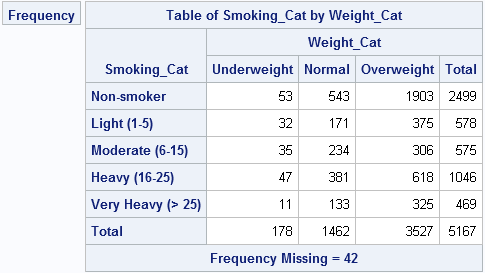By using this technique, you can specify any order for the categories of a contingency table. The technique extends to other analyses as well.

For more on using PROC FORMAT for data analysis, see the following articles:If you've ever tried to use PROC FREQ to create a frequency table of two character variables, you know that by default the categories for each variable are displayed in alphabetical order. A different order is sometimes more useful. For example, consider the following two-way table for the smoking status and weight status of 5,167 patients in a medical study, as recorded in the Sashelp.Heart data set, which is distributed with SAS software:

```proc freq data=sashelp.heart; tables Smoking_Status*Weight_Status / norow nocol nopct; run;```The alphabetical order of the categories is not the best choice for these data. A better table would order the weight categories as "Underweight," "Normal," and "Overweight." Similarly, the smoking categories should be ordered from 'Non-smoker' to "Very Heavy (> 25)."

In most introductory SAS courses—and at almost every SAS conference—you will hear recommendations about how to encode ordinal variables when you create a SAS data set. Two common ways to make specify the order of categories are:

• Create (or sort) the data in the order that you want the frequency table to appear. Use the ORDER=DATA option on the PROC FREQ statement to instruct the procedure that it should order categories as they appear in the data set.
• Encode the data as a numerical variable with values 1, 2, 3, ..., and use a SAS format to provide the text that will be displayed for each category. The FREQ procedure will order the (numerical) variables by 1, 2, 3, ..., but will display the formatted values as the headers for the frequency tables.

The first approach is simple, but it has limitations. Often the data set is handed to you; you don't get to create it. You can use PROC SORT to create a sorted copy of the data, but you still need to provide variables with values such as 1, 2, 3, ..., in order to sort the data. Furthermore, if some joint categories are empty (for example, there are no underweight non-smokers in the data), then the ORDER=DATA option might not accomplish what you want. (However, a workaround is to create a weight variable and using the ZEROS option in the WEIGHT statement to include empty levels.)

The second approach requires that you add sorting variables to the data set and apply a format to those variables. First, define the format:

```proc format; value WtFmt 1 = 'Underweight' 2 = 'Normal' 3 = 'Overweight'; value SmFmt 1 = 'Non-smoker' 2 = 'Light (1-5)' 3 = 'Moderate (6-15)' 4 = 'Heavy (16-25)' 5 = 'Very Heavy (> 25)'; run;```

Next, create new sorting variables and apply the formats to "recreate" the original variables:

```data Heart / view=Heart; format Smoking_Cat SmFmt. Weight_Cat WtFmt.; set sashelp.heart; select (Weight_Status); when ('Underweight') Weight_Cat=1; when ('Normal') Weight_Cat=2; when ('Overweight') Weight_Cat=3; when (' ') Weight_Cat=.; end; select (Smoking_Status); when ('Non-smoker') Smoking_Cat=1; when ('Light (1-5)') Smoking_Cat=2; when ('Moderate (6-15)') Smoking_Cat=3; when ('Heavy (16-25)') Smoking_Cat=4; when ('Very Heavy (> 25)') Smoking_Cat=5; when (' ') Smoking_Cat=.; end; run;```

I have created a data set view rather than a data set in order to save storage space, which might be important for large data sets. You can now call PROC FREQ, as follows:

```proc freq data=heart; tables Smoking_Cat*Weight_Cat / norow nocol nopct; run;```By using this technique, you can specify any order for the categories of a contingency table. The technique extends to other analyses as well.

For more on using PROC FORMAT for data analysis, see the following articles: